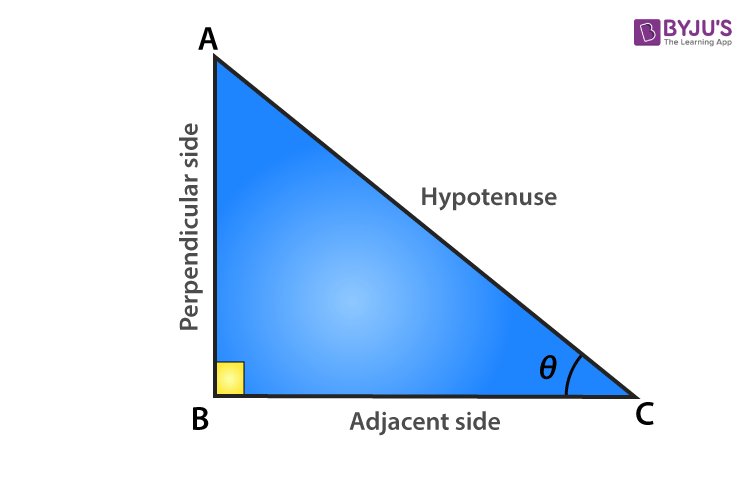Jet Set Go! All about Aeroplanes Jet Set Go! All about Aeroplanes

# Trigonometric Identities Class 10

Trigonometric identities class 10 includes basic identities of trigonometry. When we recall, an equation is considered identical, if the equations are true for all the values of variables involved. Similarly, the trigonometric equation, which involves trigonometry ratios of all the angles, is called a trigonometric identity if it is true for all values of the angles.

In Mathematics, trigonometry is one of the most important and prominent topics to learn. Trigonometry is basically the study of triangles. The term ‘Trigon’ means triangle and ‘metry’ means measurement. Trigonometric identities class 10 consists of trigonometry ratios such as sine, cosine and tangent in its equations. Even, trigonometry identities class 10 formulas are based on these ratios. These identities are used to solve various trigonometry problems.

By considering a right-angled triangle, trigonometry identities class 10 lists could be figured out. The trigonometric identities or equations are formed using trigonometry ratios for all the angles. Using trigonometry identities, we can express each trigonometric ratios in terms of other trigonometric ratios, and if any of the trigonometry ratios value is known to us, then we can find the values of other trigonometric ratios. We can also solve trigonometric identities class 10 questions, using these identities as well.

## Trigonometric Identities for Class 10

Trigonometric identities are the equations that include the trigonometric functions such as sine, cosine, tangent, etc., and are true for all values of angle θ. Here, θ is the reference angle taken for a right-angled triangle. In class 10th, there are basically three trigonometric identities, which we learn in the trigonometry chapter. They are:

1. Cos2 θ + Sin2 θ = 1
2. 1 + Tan2 θ = Secθ
3. 1 + Cotθ = Cosecθ

Here, we will prove one trigonometric identity and will use it to prove the other two. Take an example of a right-angled triangle ΔABC.Proof of Trigonometric Identities Class 10

In a right-angled triangle, by the Pythagorean theorem, we know,

(Perpendicular)2 + (Base)2 = (Hypotenuse)2

Therefore, in ΔABC, we have;

AB2 + BC2 = AC….. (1)

Dividing equation (1) by AC2, we get,

$$\begin{array}{l}\frac{AB^2}{AC^2} + \frac{BC^2}{AC^2}= \frac{AC^2}{AC^2}\end{array}$$

$$\begin{array}{l}(\frac{AB}{AC})^{2} +(\frac{BC}{AC})^{2} = (\frac{AC}{AC})^{2}\end{array}$$

$$\begin{array}{l}(cos\ \theta )^{2}+ (sin\ \theta )^{2}= 1\end{array}$$

Cos2 θ + Sin2 θ = 1 …..(2)

If θ = 0, then,

• Cos2 0 + Sin2 0 = 1
• 12 + 02 = 1
• 1 + 0 = 1
• 1 = 1

And if we put θ = 90,then

• Cos2 90 + Sin2 90 = 1
• 02 + 12 = 1
• 0 + 1 = 1
• 1 = 1

For all angles, 0°≤ θ ≤ 90°, equation (2) is satisfied. Hence, equation (2) is a trigonometric identity.

Again, divide equation (1) by AB2, we get

$$\begin{array}{l}\frac{AB^2}{AB^2} + \frac{BC^2}{AB^2} = \frac{AC^2}{AB^2}\end{array}$$

$$\begin{array}{l}(\frac{AB}{AB})^{2} + (\frac{BC}{AB})^{2} = (\frac{AC}{AB})^{2}\end{array}$$

1 + Tan2 θ = Secθ …..(3)

If θ = 0, then,

• 1 + tan20 = sec20
• 1 + 02 = 12
• 1 = 1

And if we put θ = 90,then

• 1 + tan290 = sec290
• 1 + ∞ = ∞
• ∞ = ∞

As you can see, the values of both sides are equal. Therefore, it proves that for all the values between 00 and 900, the equation (3) is satisfied. So, it is also a trigonometric identity.

Let’s see what we get if we divide equation (1) by BC2, we get,

$$\begin{array}{l}\frac{AB^2}{BC^2} + \frac{BC^2}{BC^2} = \frac{AC^2}{BC^2}\end{array}$$

$$\begin{array}{l}(\frac{AB}{BC})^{2} + (\frac{BC}{BC})^{2} =(\frac{AC}{BC})^{2}\end{array}$$

Cotθ + 1 = Cosec2 θ ….(4)

Now let us prove this identity as well.

If θ = 0, then equation (4) can be written as;

• Cot20 + 1 = Cosec20
• ∞ + 1 = ∞
• ∞ = ∞

Both sides are equal.

And if θ = 90,then equation (4) can be written as;

• Cot290 + 1 = Cosec290
• 02 + 1 = 12
• 1 = 1

Thus, proved that equation (4) is a trigonometric identity.

### Video Lesson on Trigonometry## Practice Questions From Class 10 Trigonometry Identities

1. Prove √(sec θ – 1)/(sec θ + 1) = cosec θ – cot θ
2. Prove (tan θ + sec θ – 1)/(tan θ – sec θ + 1) = (1 + sin θ)/cos θ
3. Prove sec θ√(1 – sin2 θ) = 1
4. Given, √3 tan θ = 3 sin θ. Prove sin2 θ – cos2 θ = 1/3
5. Evaluate cosθ tanθ + tanθ sinθ in terms of tan θ.